# Factors of production in the short run and in the long run

Talk to people who own or manage businesses, and it won’t be long before the word cost comes up. People in business worry about measuring costs, controlling costs, and—most of all—reducing costs. This is not surprising: Owners want their firms to earn the highest possible profit, and costs must be subtracted from a firm’s revenue to determine its profit. We will postpone a thorough discussion of profit until the next chapter. Here, we focus on just the costs of production: how economists think about costs, how costs are measured, and how they change as the firm adjusts its level of output.

If a firm wants to increase production, it will take time to obtain a greater quantity of certain inputs. For example, a producer can use more electricity by turning on more switches, but it may take a long time to buy and install more machines, and longer still to build another factory.

If the firm wants to increase output in a hurry, it will only be able to increase the quantity of certain inputs. It can use more raw materials, more tools and possibly more labour. But it will not be able to build a new building or buy additional machines. Thus the factors of production used by the firm can be classified into fixed factorsandvariable factors. If a firm cannot obtain more of acertain factor within the period under consideration, the factor is said to be a fixed factor. If the factors can be varied, they are called variable factors. The distinction between fixed and variable factors allows us to distinguish between the short run and the long run.

When we speak of the short run and the long run, we are not referring to definite periods of calendar time. We are referring to certain conditions. We define the short run and the long run in terms of fixed and variable factors. The short run is a situation in which the firm has at least one fixed factor, while the long run is a situation in which all the firm’s factors are variable.

The short run for the firm is a time period when at least one of the production resources (factors) of the firm is fixed. Usually this is fixed capital of the firm. In the short run a firm can change the intensity of using fixed factor by varying the quantities of other, variable factors (in particular, labor).

The long run for the firm is a period when it can vary quantities of all resources it uses, including fixed capital.

 In the short run there are variable resources and fixed resources and there are two types of costs - variable costs and fixed costs. Variable cost (VC) is the cost of inputs that change with output. Fixed cost (FC) is the cost of inputs that are independent of output (The common business term for FC is overhead cost). Total cost (TC) is the sum of the cost of all inputs used to produce a good. Total cost in the short run are equal to the sum of variable and fixed costs at the given volume of output. All types of costs (excluding fixed costs) depend on the output Q: VC=g(Q), TC = c(Q) = FC + g(Q).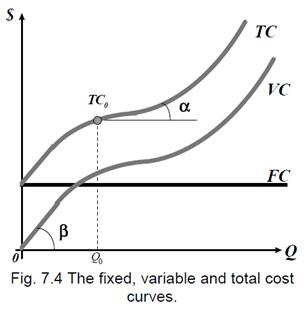Average cost (AC, ATC) is the total cost per unit of output AC = TC / Q.

Average variable cost (AVC) is the variable cost per unit of output AVC = VC / Q.

Average fixed cost (AFC) is the fixed cost per unit of output

AFC = FC / Q. AC = AFC + AVC.

Marginal cost (MC) is the change in total cost (ΔTC) that results from a change in output (ΔTC); the extra cost incurred to produce another unit of output MC = ΔTC / ΔQ;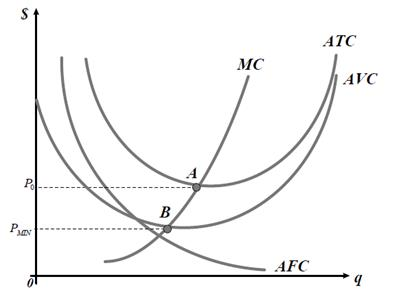Q, ед Cost, (тенге) FC VC TC MC AFC AVC ATC 0 1 2 3 4 5 6 7 50 50 50 50 50 50 50 50 0 36 50 80 104 140 196 300 50 86 110 130 154 190 246 350 - 36 24 20 24 36 56 104 - 50,0 25,0 16,7 12,5 10,0 8,3 7,1 - 36,0 30,0 26,7 26,0 28,0 32,7 42,9 - 86,0 55,0 43,3 38,5 38,0 41,0 50,0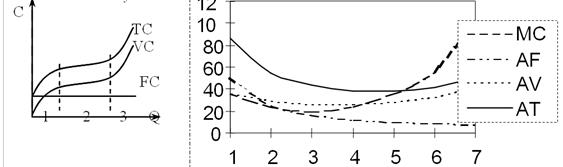2. Classification and structure of costs of the firm

In the long-term period all desirable changes in quantity and structure of all resources can be carried out both separate firm, and branch as a whole. For this period the firm can change capacity of the equipment, its quantity, master a new kind of production or even at all to leave from the market. Therefore here all resources are variables. And the firm chooses that which will allow it to make production with the minimal average costs from available technologies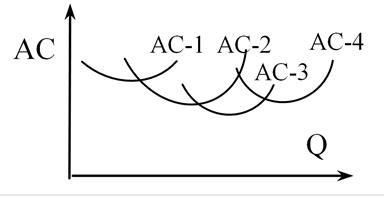Firms seek to maximize their profits, i.e. they attempt to produce as cheaply as possible. Thus, profit (π)can be defined in terms of revenue and costs. Revenue (R)is what the firm earns by selling goods or services in a given period such as a year. Costs (C)are the expenses which are necessary for producing and selling goods or services during the period. Profit is the total revenue (TR) from selling the output minus the total costs (TC) of inputs used:

π = TR – TC

Economic Profitoccurs when total revenue exceeds total cost. The revenue of the firm more than covers all opportunity cost. After paying the explicit cost and accounting for the implicit cost, the firm has revenue left over. This remaining revenue is economic profit.

The normal profit - the minimal payment necessary to keep enterprise ability within the limits of the given activity. If compensation for enterprise work will appear below this size that the businessman will be switched to other, more attractive activity.

Profit is an absolute indicator that illustrates how efficient the production of the firm is. If we measure the efficiency of costs per a unit of output, we will know how profitable the firm is, in other words, the profitability= profit / costs. For example, if the profits are 15 money units and costs are 100 money units, then the profitability is 0.15 (15%).

Marginal revenue (MR)is the corresponding change in total revenue from selling one more unit of output.

The actual length of the short run will differ from firm to firm. If it takes a farmer a year to buy new land, buildings and equipment, the short run is any time up to a year, and the long run is any time period longer than a year. On the other hand, if it takes a shipping company three years to obtain an extra ship, the short run is any

period up to three years, and the long run is any period longer than three years.

Firms seek to maximize their profits, i.e. they attempt to produce as cheaply as possible. Thus, profit (π) can be defined in terms of revenue and costs. Revenue (R) is what the firm earns by selling goods or services in a given period such as a year. Costs (C) are the expenses which are necessary for producing and selling goods or services during the period. Profit is the total revenue (TR) from selling the output minus the total costs (TC) of inputs used:

π = TR – TC where π a Greek letter ‘pi’represents profits.

Profit is an absolute indicator that illustrates how efficient the production of the firm is. If we measure the efficiency of costs per a unit of output, we will know how profitable the firm is, in other words, the profitability = profit / costs. For example, if the profits are 15 money units and costs are 100 money units, then the profitability is 0.15 (15%).

Costs (C)are the expenses which are necessary for producing and selling goods or services during the period.

Costs should include opportunity costs of all resources used in production. Opportunity cost of a commodity is the amount obtained by an input in its best alternative use. In particular, costs include the owner’s time and effort in running the business. Costs also include the opportunity cost of the financial capital used in the firm.

Factors not owned by the firm are called explicit costs. They are direct payments to outside suppliers of inputs, for example, payments for electricity. Factors already owned by the firm are called implicit costs. They are the costs that do not involve a direct payment of money to a third party, but which nevertheless involve a sacrifice of some alternative. For example, the firm owns machinery and it does not have to pay for using them. Their opportunity costs are thus implicit costs.

Aiming to get higher profits, firms obtain each output level as cheaply as possible. Firms choose the optimal output level to receive the highest profits. This decision can be described in terms of marginal cost and marginal revenue.

Marginal cost (MC)is an increase in total cost when oneadditional unit of output is produced.

Marginal revenue (MR)is the corresponding change in totalrevenue from selling one more unit of output.

As the individual firm has to be a price-taker, each firm’s marginal revenue is the prevailing market price. Profits are the highest at the output level at which marginal cost is equal to marginal revenue, that is, to the market price of the output. If profits are negative at this output level, the firm should close down.

An increase in marginal cost reduces output. A rise in marginal revenue increases output. Of course, the optimal quantity supplied is affected by such noneconomic factors as technology, environment, etc.

Other cost conceptsare average cost (AC) and averagetotal cost (ATC), average fixed cost (AFC) and average variable cost (AVC). If Q represents the quantity of output produced, these concepts can be defined as follows:

AFC = TFC/Q: AVC = TVC/Q; ATC = TC/Q; ATC = AFC + AVC

3. Financial position and income of the firm. Terms maximize the firm's profits.

However, under the capital as object of sale and purchase in the market, usually mean all stock of the capital blessings available in economy, and the stream of the new capital appearing during the given period on manufacture. Thus desirable change of a stock of the capital is planned investments.

As a whole in economy of firm show demand not simply, on what that material benefits (machine tools, the equipment и.т.п.), and on temporarily free money resources which can be spent for purchase of these material benefits and to return, having given a part of profit on their use in the future. Therefore demand for investments is a demand for extra means, and loan percent - the price of extra means. Therefore when it is a question of the market of the capital the market of the monetary capital means. Tools of this market can be both directly credit resources, and securities and any sort derivative contracts.

The size of demand for the monetary capital decreases with growth of the rate of percent. As all is equal to owners of the monetary capital where to put means (adjusted for risk), the market provides alignment of profitableness of capital investments in the uniform rate of percent. Thus the principle of alternative costs dictates necessity to provide profitableness not below the given rate of percent.

The decision of a problem of comparison of expenses and incomes in the future is in the present by discounting - operation of reduction of the future incomes and charges by the uniform (initial) moment of time. The rate of discount (r) - percent which can be received in the market for the capital given on credit, possible rate of return on the invested capital or a discount rate of percent (the rate on which the Central bank gives out loans to commercial banks). The choice of the rate of discount depends on what alternative ways of use of the capital are accessible to the given agent, therefore r it is possible to consider as rate of return which can be received from best of all alternative ways of capital investment. Thus, today's value (m0) the monetary income (mt) which will be received through t years will make:

 m0 = mt (1 + r)t

For an estimation of perspectivity of various directions of capital investments use following parameters: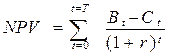Where t - Current, from the beginning of realization of the project, year;

Т - Full term of realization of the investment project, years;

Bt - The income received by firm from realization of the project in year t, tenge;

Сt - Charges of firm on realization of the investment project in a year t, tenge;

r - The rate of discounting in that to year.

Investments will be justified if NPV > 0.

 Theme № 9. Markets of factors of production and income distribution. Lecture 9

1. The labor market and its essence and characteristics. Demand and supply of labor. The essence and form of salary.
2. The capital market and its structure. Capital as an investment. The circuit and the turnover of capital.
3. The land market. Demand and supply of land. Land rent. The price of land.

Дата добавления: 2015-10-05; просмотров: 1067; ЗАКАЗАТЬ НАПИСАНИЕ РАБОТЫ

Поиск по сайту:

При помощи поиска вы сможете найти нужную вам информацию, введите в поисковое поле ключевые слова и изучайте нужную вам информацию.

Поделитесь с друзьями:

Если вам понравился данный ресурс вы можете рассказать о нем друзьям. Сделать это можно через соц. кнопки выше.
helpiks.org - Хелпикс.Орг - 2014-2021 год. Материал сайта представляется для ознакомительного и учебного использования. | Поддержка
Генерация страницы за: 0.016 сек.